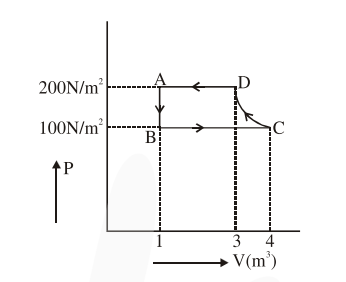# The P-V diagram of a diatomic ideal gas system goingQuestion:

The P-V diagram of a diatomic ideal gas system going under cyclic process as shown in figure. The work done during an adiabatic process CD is (use $\gamma=1.4$ ) :1. $-500 \mathrm{~J}$

2. $-400 \mathrm{~J}$

3. $400 \mathrm{~J}$

4. $200 \mathrm{~J}$

Correct Option: 1

Solution:

Adiabatic process is from $C$ to $D$

$\mathrm{WD}=\frac{\mathrm{P}_{2} \mathrm{~V}_{2}-\mathrm{P}_{1} \mathrm{~V}_{1}}{1-\gamma}$

$=\frac{200(3)-(100)(4)}{1-1.4}$

$=-500 \mathrm{~J}$